http://people.csail.mit.edu/jaffer/SimRoof/Convection/Measurements

# Measurements of Convection From a Rectangular Plate

See my blog for the history of the Convection Machine project.

## Anemometer

To test the theoretical predictions, accurate measurements must be made with no fewer than two different air velocities. I found three commercial hot-wire anemometers:

TPI 565C1 Hotwire Air Velocity Meter \$170
This unit has ±(5% of reading + 3 digits) for its 0.2 to 20.0m/s range.
That is ±12.5% at 4 m/s; ±15.0% at 3 m/s; ±20.0% at 2 m/s; and ±35.0% at 1 m/s.
Amprobe TMA20HW Hot Wire Anemometer \$210
This unit has ±(3% reading + 1% full-scale) for its 0 to 30.0m/s range.
That is ±10.5% at 4 m/s; ±13.0% at 3 m/s; ±18.0% at 2 m/s; and ±33.0% at 1 m/s.
Thomas Traceable Digital Hot Wire Anemometer, with RS-232 Output \$480
This unit has ± (1% of full scale + 1 digit) for its 0 to 20.0 m/s range.
That is ±7.5% at 4 m/s; ±10.0% at 3 m/s; ±15.0% at 2 m/s; and ±30.0% at 1 m/s.

At the airflow velocities attainable by my wind-tunnel, the error tolerances of these anemometers are inadequate.

A small impeller-based anemometer (which also claims NIST traceability) turns out to have better accuracy over the range of interest:

Kestrel 1000 Wind Meter \$74
This unit has ± Max(3% of reading, 1 digit) for its 0.6 to 40.0 m/s range.
That is ±3.0% at 4 m/s; ±3.3% at 3 m/s; ±5.0% at 2 m/s; and ±10.0% at 1 m/s.

Ambient Weather has a similar unit, but with incomplete accuracy specifications.

Ambient Weather WM-2 Handheld Weather Meter \$26
This unit claims ± 3% of reading for its 0.4 mph to 67 mph range (0.2 to 30.0m/s).

## TunnelThe primary requirement for the test chamber is that it supply uniform airflow across the plate. Conventional wind tunnels are designed to supress turbulence, which is not a requirement here. A tunnel with a 35.6 cm × 61 cm cross section (and 61 cm length) has been built which allows the plate assembly to be centered with 15 cm of space on all sides. The specification of the 0.5 m diameter fan implies that, free of obstructions, it should be able move air at over 15 m/s through the tunnel. Pulling air through the tunnel, however, a maximum of 4.1 m/s has been achieved, and this had negligible effect on the fan outflow speed.

The hydraulic diameter for the tunnel DH=44.9 cm. A 10 m/s flow through the tunnel length of 61 cm would drop only 3 Pa of pressure (by the Darcy–Weisbach equation). At 3.7 m/s, the pressure drop is calculated to be .421 Pa.

The cross-section of the test chamber is .217 m2. When the plate is in the tunnel, it will reduce its effective cross-section, causing the air to flow faster through the restricted cross-section. Thus a 30.5 cm × 30.5 cm × 2.5 cm plate reduces the cross-section by .0077 m2 (3.6%), increasing the 3.6 m/s flow by 3.6% to 3.73 m/s. An increase of around 0.1 m/s was observed, although that is also the limit of the anemometer's resolution.

The plate with its back insulated will have a cross section of 0.13 m2, increasing flow by 7% to 3.85 m/s.

With some improvements to the fan cowling and using an auto-transformer to overdrive the fan, speeds of 4.6 m/s have been achieved with the plate in the tunnel.

## Plate

Corresponding to the dimensionless numbers in the table in Experiments, the forced convective heat-flow-to-temperature-difference ratios for 21°C air with 40% relative humidity flowing by a 30 cm square plate at 26°C are:

ε V h⋅A V h⋅A V h⋅A 3.0mm 1.02m/s 1.08W/K 2.05m/s 2.17W/K 4.09m/s 4.33W/K 1.5mm 1.02m/s 0.819W/K 2.05m/s 1.64W/K 4.09m/s 3.27W/K 0.0m 1.02m/s 0.708W/K 2.05m/s 1.23W/K 4.09m/s 2.15W/K

The plate must be heated or cooled in order for there to be convective heat transfer to measure. Thermal radiation from a plate can be significant. A 1K temperature difference at 21°C ambient temperature can transfer 5.3 W/(K⋅m2) between 95% emissive surfaces, which dwarfs the natural convection for the plate. The emissivity of the plate and its surroundings must be estimated and compensated for; but using low emissivity materials will improve accuracy overall. A plate machined from aluminum will have emissivity around 8%. Combined with an enclosure with 95% emissivity, this results in 40 mW/(K⋅m2) of radiative transfer.

The back of the plate will be covered by thermal insulation. polyisocyanurate has a thermal conductivity around .023 W/(K⋅m). Through a 30.5 cm×30.5 cm×2.54 cm board its thermal conductance is .084 W/K.

The heated or cooled plate can also experience natural convection. The natural convective and radiant heat flows for a plate with temperature-differences of 1K, 5K, 10K, and 15K are:

 ΔT h⋅A⋅ΔT ΔT h⋅A⋅ΔT ΔT h⋅A⋅ΔT ΔT h⋅A⋅ΔT upward 1K 0.239W 5K 1.83W 10K 4.42W 15K 7.41W vertical 1K 0.169W 5K 1.33W 10K 3.24W 15K 5.47W downward 1K 84.8mW 5K 0.585W 10K 1.34W 15K 2.19W radiant 1K 39.5mW 5K 0.197W 10K 0.395W 15K 0.593W

While forced convection, radiant transfer, and backside leakage grow linearly with increasing temperature difference, natural convection grows faster. To minimize the effect of natural convection on forced convection, experiments should use the smallest temperature difference which can be accurately measured (and larger than the variation across the surface) and have the heated convective surface face down.

The expected forced convective surface conductance for a 5 K temperature difference is:

ε V h⋅A⋅ΔT V h⋅A⋅ΔT V h⋅A⋅ΔT 3.0mm 1.02m/s 5.42W 2.05m/s 10.8W 4.09m/s 21.7W 1.5mm 1.02m/s 4.09W 2.05m/s 8.19W 4.09m/s 16.4W 0.0m 1.02m/s 3.54W 2.05m/s 6.16W 4.09m/s 10.7WPictured to the right is the 30 cm × 30 cm apparatus for measuring the convection from its rough surface. Machined from MIC-6 aluminum to keep its emissivity low, the shiny surface is composed of 8.28 mm × 8.28 mm × 6 mm posts spaced on 11.7 mm centers. The area of the top of each post is .686 cm2, half of the 1.37 cm2 cell. The average (also the root-mean-squared) height of roughness is thus 3 mm.The beveled back surface has 9 electric heating elements attached. The heating elements are recessed so that they don't come into contact with the thermal insulation covering the back side. The view to the right shows the heating elements (TO-220 resistors) in their cavities cut in the back of the plate. The gray ribbon cable connects to an electronic sensor in a blind hole to measure the plate's temperature. Electronic circuitry will activate the heating elements as needed to maintain the plate temperature at 5K above the ambient air temperature (as measured by an external sensor). At thermal equilibrium, the electrical power consumed by the heating elements will be the heat flow, which will be mostly due to convection.

The full scale convective surface conductance at V=4 m/s and ΔT=5 K is expected to be 34 W. So the heating elements should be able to supply in excess of 50 W of heat to avoid long settling times.

MIC-6 aluminum's bulk thermal conductivity is 142 W/(K⋅m). The plate's thermal conductance over its full thickness (2.2 cm) is 600 W/K. That is, 1K of temperature drop will result from every 600 W of heat flow. The thermal conductance from the bisecting plane to the edge (15 cm) is 6.25 W/K. As long as heaters are distributed well over the backside, the thermal resistance of the plate itself can be ignored. Aluminum's specific heat is .897 J/(g⋅K), its density 2.70 g/cm3, yielding a thermal capacity of 4.69 kJ/K for the slab. 50 W will heat the slab at the rate of 1 K per 78 s.

The weight of the plate will be 5.2 kg. How will it be supported in the wind tunnel? If it were upward facing, it could be stacked on blocks. But with a temperature difference of 5 K, an upward facing plate has 3 times the natural convection of a downward facing plate. Fashioning a rigid thermally insulated support which doesn't affect the air flow seems a daunting task. Instead, it can be suspended from 4 mono-filament (fishing) lines looped around the 4 corner posts of the downward facing plate which are wound on zither tuning-pins at 8 points above it in the wind tunnel. The corner post edges may need to be rounded in order to avoid severing the lines.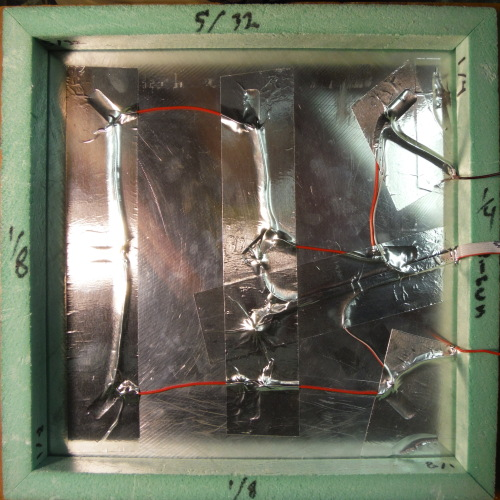The photo to the right shows version two of the back of the plate. The voids and recesses are covered with aluminum tape. The extruded polystyrene foam wedges are glued to the bevel to achieve a better seal than version one had. The goal of version two is make a configuration of insulation which can be mathematically modeled.The photo to the right shows the back with the square of polyisocyanurate foam in place. The gray wires connect to a temperature sensor. The temperature sensor in contact with the foil facing makes it possible to measure the heat conduction through the foam.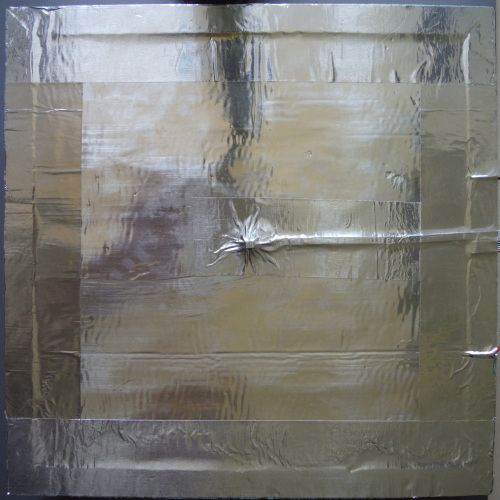Here is its final form with aluminum tape extending the foil to the whole back plane.

## ElectronicsLogging of the measurements is performed by a STMicroelectronics - ARM STM32F3 Discovery 32-Bit ARM M4 72MHz development board. The larger board, which it plugs into, contains power supplies, heater control and drive, signal conditioning, and a three-digit 7-segment display, here showing the fan rotational speed as 67 r/min. The 7-segment displays are disabled during data collection in order to minimize electrical noise in the microprocessor's analog-to-digital converters.

Data is captured in the microprocessor's non-volatile RAM once a second for 108 minutes per run. After the run is complete I transfer the data over a USB serial connection. The black USB cable is seen at the top right of the image.

The two yellow blocks connected by black cables to the board are receptacles for Lenovo laptop 20.5 volt power supplies. The large black object in the lower right is a heat sink for the heater drive transistor. Glued to the heater drive transistor is a temperature sensor; my logger program shuts the board down if the transistor temperature exceeds 125°C.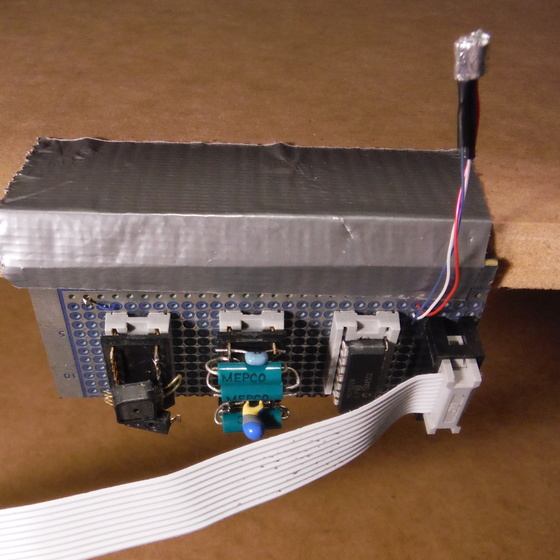This small board measures pressure, humidity, and temperature of the air at the wind-tunnel intake. The LM35 temperature sensor sticks up into the wind-tunnel test chamber; it is wrapped in aluminum tape so that radiative transfer is minimized. The temperature sensor is powered only while it is being read so that self-heating doesn't affect it; self-heating was a problem before it was switched.

The air-pressure sensor is a black block on the left side. The humidity sensor is below the air-pressure sensor.The image to the right is linked to the schematics in PDF form.

## Plate in Tunnel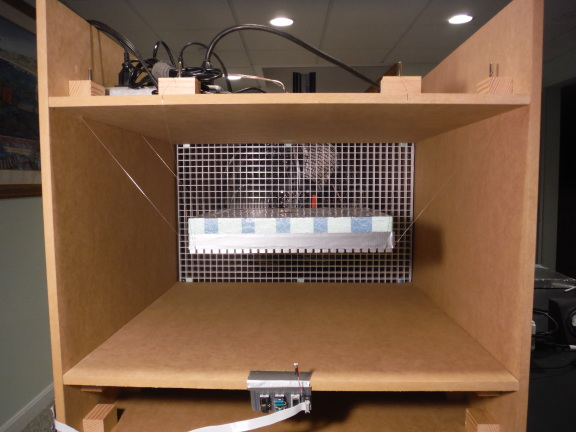Martin Jaffer helped suspend the plate in the wind tunnel. The plate is suspended from four 0.38 mm-diameter lengths of steel piano wire terminated at eight zither tuning pins in wooden blocks above the test chamber. The wire is sheathed by close-fitting Teflon tubing where it would contact the plate. The plate is not centered, but shifted halfway towards the front of test chamber. This gives more clearance from the turbulent transition regions spreading from the tunnel walls.

There is a strip of duct-tape covering the metal edge between the insulation and the machined surface. The ambient sensor board (temperature, pressure, humidity) can be seen at the bottom edge of the tunnel.This photo shows the electronics board and cabling.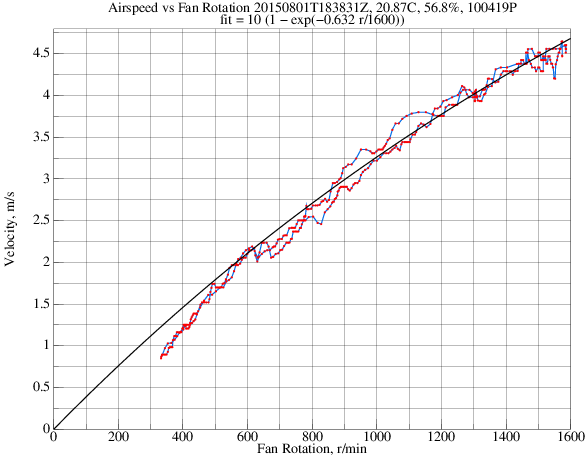Interrupt-driven code measures the rotation rate of the fan. Each second it divides the number of times a blade has passed the sensor by the length of time between the zeroth and last blade passing. This reduces quantization error such that most readings are stable to within a couple of r/min.

The graph to the right shows a calibration run linking anemometer measurements with fan rotation rate. The graph is linked to a pdf of 5 calibration runs taken at different times. It is more important to fit the curve to the high speeds than the low because the anemometer accuracy drops with decreasing speed. This is discussed in more detail in Fan Calibration.

## Modeling the Other Sides

Measurements are always of heat flow from all sides of the plate. To infer the non-test surfaces from measurements of total heat flow would require that the heat flow from the front of the plate be precisely known. But the point of this experiment is to measure the heat flow from the front.

An insulating cover for the front of the plate turned out to interact strongly with the convection from the short sides, so we can't measure our way to a solution. The only option remaining is to analyze and estimate the heat flow through the back of the plate.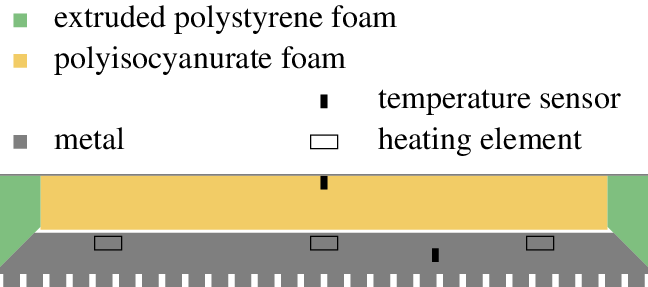Consider the square back and the sides separately. The square back is primarily composed of a 0.267 m × 0.267 m × 25.4 mm block of polyisocyanurate foam insulation. Both sides of the foam insulation are faced with aluminum foil. At the center of the external foil is a temperature sensor. The foil facing is fortuitous because it equalizes the temperature across each side, turning the foam's thermal behavior into a case of conduction of a solid material between two parallel isothermal plates. Adjoining the perimeter of the polyisocyanurate foam block are four 19 mm wide extruded polystyrene foam pieces. Aluminum tape extends the outer facing to 305 mm, the full width of the test plate. In the cross-section above, the trunacted pyramid to be analyzed first is enclosed in a dotted line.

Heat flowing from the test plate through the polyisocyanurate foam causes convective heat transfer from the external facing. Considered as lumped elements, the convective surface conductance is in series with the foam conductance and the conductance of 1 mm airspace between the test plate and the polyisocyanurate foam. Conductances in series are computed as the reciprocal of the sum of the reciprocals of the individual conductances.

The thermal conductance through a 0.267 m × 0.267 m × 25.4 mm block of polyisocyanurate foam is 64.5 mW/K. What is needed is the conductance through a truncated pyramid where the top is 0.267 m × 0.267 m and the bottom is 0.305 m × 0.305 m.

It is instructive to calculate the thermal conductance of the foam rolled into a pipe. The outer radius ro is 25.4 mm longer than inner radius ri. We want an angle θ to subtend the rolled foam such that θri=.267 and θro=.305. That θ is 3/2. The conduction through a .267 m long pipe is then:

 θ 0.023 W/(m⋅K) 0.267 m ln (ro / ri) = 69.0 mW/K

Compare this with the conductance of a block having dimensions of 0.267 m by the geometric mean of 0.267 m and 0.305 m: 69.0 mW/K. It is within 0.01% of the pipe conductance!

Using the geometric mean for both sides, the thermal conductance of the truncated pyramid is 73.7 mW/K. 64.5 mW/K of that is due to the rectangular polyisocyanurate foam block. The rest is through extruded polystyrene foam. Multiplying the difference (9.21 mW/K) by the ratio of conductivities and adding that to 64.5mW/K gives the conductance of 78.1 mW/K for the truncated mixed material pyramid.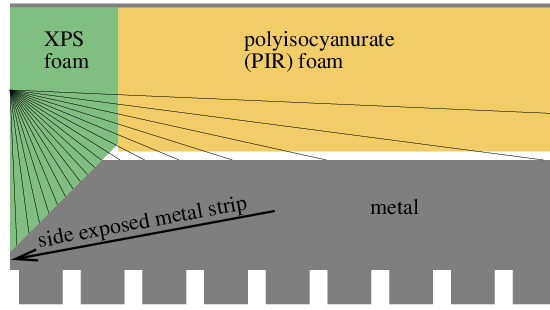The vertical side is not an isothermal surface. There are exposed metal post sides, a 4 mm metal strip covered by duct-tape, and a wedge of extruded polystyrene insulation. Because the vertical side is not isothermal, each point's effective surface conductance will depend on the temperature profile along the side. So an exact solution is not possible.

A point on the side which is near to metal will have a large conductance. So summing the conductivity divided by distance to metal at each angle will be roughly proportional to the local conductance. The conductance through a slab with parallel isothermal faces is well known. The ratio of the slab conductance to the slab sum is the factor (0.637) which will normalize conductivities calculated this way.

With forced air the four sides behave differently. The forced air flows parallel to the long dimension on two sides but flows into the windward side and away from the leeward side.

The forced convective component for the two parallel sides averages the local forced convective surface conductance (assuming uniform wind speed) in series with that point's insulation conductance as computed above.

For the windward side, a similar calculation is done, but the wind speed grows linearly from 0 at the mid-line to V along each long edge. For the leeward side the wind speed grows linearly from 0 along each long edge to V at the mid-line, but with the flow always turbulent. The leeward surface conductance turned out to be within 1% of the windward surface conductance, so double the windward value is used. These local surface conductance integrations consume most of the simulation time.

For the natural convection component, theory doesn't address non-isothermal surfaces. However, the wedge conductivity drops quickly as one moves away from the 4 mm metal strip. So I calculate the natural convection from the 4 mm metal strip (which is isothermal) extended by the average height of posts: 0.006/sqrt(2). The actual surface conductance could be larger, because of convection from the wedge, or smaller, because air must flow along the wedge to reach the metal strip. So I included a "fudge factor" in the side natural convection calculations.

The radiative heat flow from the 4 mm metal strip covered by duct-tape will dwarf the flow from the bare metal posts and foam wedge, so it alone is modeled along with a separate fudge-factor. While heat conduction through the foam wedge will increase the fudge factor, heat rising from the downward convecting surface should not be counted twice.

In the absence of measurement, 0.8 is used as the emissivity of the duct-tape; 0.8 for the wind-tunnel and surroundings visible to the plate. With convective and radiative fudge factors of 4.01 and 0.41 respectively, the unaccounted for natural convection for both upward and downward facing rough surfaces are nearly equal to that expected from a smooth surface.

## Integration

The physical quantities to be measured are:

symbol units description TF K Fluid (Air) Temperature TP K Plate Surface Temperature P Pa Fluid (Atmospheric) Pressure V m/s Fluid Velocity Φ Pa/Pa Relative Humidity ΠH W=J/s Heater Power

Previous laboratory measurements of forced convection have been performed by starting the fluid flow and plate heater, waiting until the system reaches equilibrium (as indicated by a stable plate temperature), then recording the measurements of the physical quantities.

Because the heat flow for forced convection over a rough surface is expected to be larger than for a smooth surface, our test plate is more massive than previous experiments in order to maintain uniform temperature across the plate. This results in settling times of minutes in the best case and times approaching and hour at low airflow rates.

Radiative heat transfer is not directly measurable separately from convective heat transfer. The approach here is to minimize radiative transfer by making the test plate and its insulated backside have low emissivity (roughly 8%). The hR of the plate is estimated assuming that the emissive surfaces (mostly the inside of the wind-tunnel) are at ambient temperature. The insulated backside will be at a lower temperature than the plate. With a 5K temperature difference between the plate and ambient, the backside is about 1K above ambient in still air; in moving air this difference will be less. The small temperature difference the backside and ambient, combined with its low emissivity mean that its radiative transfer can be lumped with the backside convection UB(V).

The equation of state for the plate in forced convection is:

 ΠH = h(V) ⋅ AP ⋅ (TP − TF) + εP ⋅ εT ⋅ hR ⋅ AP ⋅ (TP − TF) + UB(V) ⋅ (TP − TF) + C ⋅ dTP dt
symbol value units description C 4760 J/K Plate Thermal Capacity AP 0.090 m2 Convecting Area εS 0.08 Plate Surface Emissivity εT 0.80 Tunnel Surface Emissivity hR 5.87 W/(m2K) Radiative Surface Conductance h(V) W/(m2K) Modeled Convective Surface Conductance UB(V) W/K Backside Surface Conductance (including radiative)

By collecting terms having a factor of (TPTF) into U(V), the equation of state is simplified:

 U(V) = h(V) ⋅ AP + εP ⋅ εT ⋅ hR ⋅ AP + UB(V)
 ΠH = U(V) ⋅ (TP − TF) + C ⋅ dTP dt
 TP(t) = TF(t) + ΠH(t) U(V) − C  U(V) ⋅ dTP(t) dt

This linear differential equation could be solved analytically, but because the independent variables are measured at discrete times, it make more sense to solve the analogous finite difference equation.

 TP(t) = TF(t) + ΠH(t) U(V) − C  ⋅ (TP(t) − TP(t')) U(V)  ⋅ (t−t')
 TP(t) ⋅ C  + U(V)  ⋅ (t−t') U(V)  ⋅ (t−t') = TF(t) + ΠH(t) U(V) + TP(t') ⋅ C  U(V)  ⋅ (t−t')
 TP(t) = ΠH(t) ⋅ (t−t') + TP(t') ⋅ C  + TF(t) ⋅ U(V) ⋅ (t−t') C + U(V)  ⋅ (t−t')

When data is sampled every second, this simplifies to:

 TP(t) = ΠH(t) + TP(t') ⋅ C  + TF(t) ⋅ U(V) C + U(V)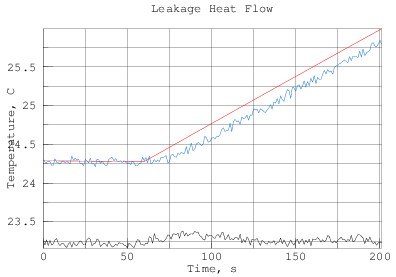I had originally planned that my controller program would servo the plate temperature in a narrow range. But when I saw the first datasets from the plate being heated, I realized that having the plate temperature slew through a temperature span enables me to separate the dynamics of heating from convection. On the basis of the heating slope I make slight adjustments to C to compensate for the addition of insulation; I won't clutter this article with that detail.

The image to the right shows the calculated TP(t) (red) versus the measured TP(t) (blue) and ambient temperature (black). Clearly there is a delay between the application of heat starting at 60 seconds and the plate temperature.Introducing a 15 second delay for ΠH makes for a much better fit.

 TP(t) = ΠH(t−15) + TP(t') ⋅ C  + TF(t) ⋅ U(V) C + U(V)

The equation of state will be solved for h(V) later. For development and test, simulation and visualization yield insights more readily than statistics.

The temperature sensor on the external face of the insulation provides the opportunity to check the insulation thermal model.

 UI ⋅ (TP − TB) = hB(V) ⋅ AP ⋅ (TB − TF) + εP ⋅ εT ⋅ hR ⋅ AP ⋅ (TB − TF) + CB ⋅ dTB dt
symbol value units description CB 45 J/K Insulation+Foil Thermal Capacity AP 0.090 m2 Convecting Area εS 0.08 Foil Surface Emissivity εT 0.95 Tunnel Surface Emissivity hR 5.87 W/(m2K) Radiative Surface Conductance hB(V) W/(m2K) Convective Surface Conductance UI 0.078 W/K Insulation Conductance

Collect surface conductance terms into UB. In natural convection UB is a function of TB, in forced convection a function of V.

 UB = hB(V) ⋅ AP + εP ⋅ εT ⋅ hR ⋅ AP
 UI ⋅ (TP − TB) = UB ⋅ (TB − TF) + CB ⋅ dTB dt
 (UB + UI) ⋅ TB(t) = UI ⋅ TP(t−110) + UB ⋅ TF(t) − CB ⋅ TB(t) − TB(t') t − t'
 TB(t) = (UI ⋅ TP(t−110) + UB ⋅ TF(t)) ⋅ (t−t') + CB ⋅ TB(t') (UB + UI) ⋅ (t − t') + CB

The 110 s time delay through the polyisocyanurate foam works both ways. The TF used through the back should be delayed 110 s. Because the actual back temperature TB is available, use it, delayed by 110 s, as input to the plate temperature simulation. That way discrepancies in the back temperature simulation don't screw up the plate temperature simulation.

 ΠH = h(V) ⋅ AP ⋅ (TP − TF) + εP ⋅ εT ⋅ hR ⋅ AP ⋅ (TP − TF) + UI ⋅ (TP − TB) + C ⋅ dTP dt
 U(V) = h(V) ⋅ AP + εP ⋅ εT ⋅ hR ⋅ AP
 ΠH = U(V) ⋅ (TP − TF) + UI ⋅ (TP − TB) + C ⋅ dTP dt
 TP(t) = ΠH(t−14) + U(V) ⋅ TF(t) + UI ⋅ TB(t−110) − C ⋅ dTP(t)/dt (U(V) + UI)

Solving the finite-difference equation:

 TP(t) = (ΠH(t−14) + U(V) ⋅ TF(t) + UI ⋅ TB(t−110)) ⋅ (t − t') + C ⋅ TP(t') (U(V) + UI) ⋅ (t − t') + C
 TP(t) = (ΠH(t−14) + U(V) ⋅ TF(t) + UI ⋅ TB(t−110)) ⋅ dt + C ⋅ TP(t−dt) (U(V) + UI) ⋅ dt + C

## Measuring

Forced convection measurements are usually presented as Nu versus Re. In order to obtain Nu, the equation of state should be solved for h(V). Because the equation of state takes the time derivative of the noisy plate temperature TP, the instantaneous value of h(V) will vary both positive and negative. But the value averaged over time should be reasonable. Where UB included both the back surface and sides before, here it is split between UI and US.

 ΠH = h(V) ⋅ AP ⋅ (TP − TF) + εP ⋅ εT ⋅ hR ⋅ AP ⋅ (TP − TF) + UI ⋅ (TP − TB) + US ⋅ (TP − TF) + C ⋅ dTP dt
 h(V) = ΠH(t−14) − UI ⋅ (TP(t) − TB(t−110)) − C ⋅ (TP(t)−TP(t−dt)) / dt AP ⋅ ((TP(t)+TP(t−dt))/2 − (TF(t)+TF(t−dt))/2) − εP ⋅ εT ⋅ hR − US / AP

The averaging of TP(t) and TP(t−dt) and also TF(t) and TF(t−dt) are needed in the denominator in order for the derivative not to correlate with the denominator, causing bias; in electronics this bias is called charge-pumping.

## Results

These first graphs show upward and downward natural convection from the plate. The four sides being vertically asymmetrical, the natural convection from them would not necessarily have the same magnitude with the rough side facing up and down. But a single value for both can be chosen such that the upward and downward convection from the rough surface matches that expected for a smooth surface.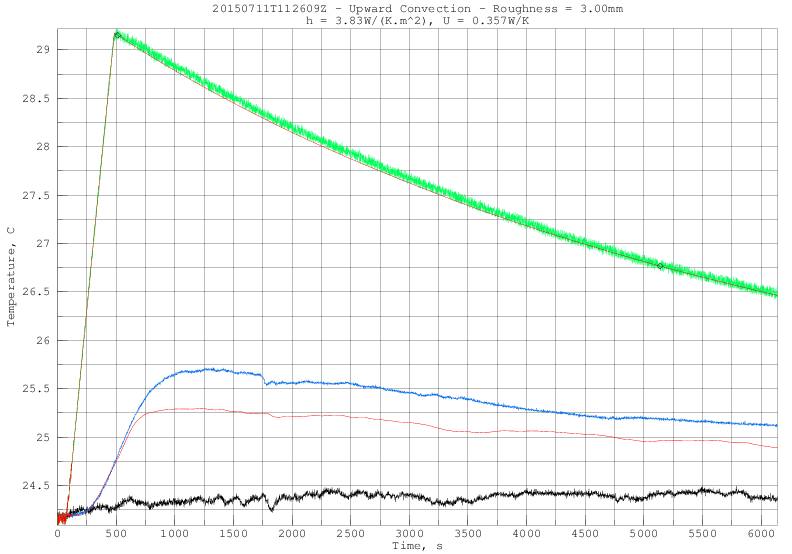The blue trace is the measured temperature from the smooth back. The simulated red trace is a good match when the smooth back is on top. The poor match below when the smooth back is facing down is probably because the plate was not suspended, but sitting on small wooden blocks without much clearance. I will perform the measurement again with more clearance in the future.This graph shows the experimental support for my "Scaled Colburn Analogy Asymptote" formula for forced convection from a rough plate. It is linked to a pdf of graphs comparing simulation with experiment. When looking at the graphs, remember that it is the slopes of the lines, not their magnitudes which are important. The measured quantities written at the top of the graphs are averaged over data between the diamond marks on the green measured plate temperature line in the individual experiments. The first diamond is 3 heat-delays after end of plate heating; the second is when the plate-ambient temperature difference drops to 1/2 of its peak value, or the end of recording, whichever comes first. The measured h, U, and Nu/Pr1/3 quantities are what remains of the total convection after reduction by the simulated heat flow from the five smooth plate faces.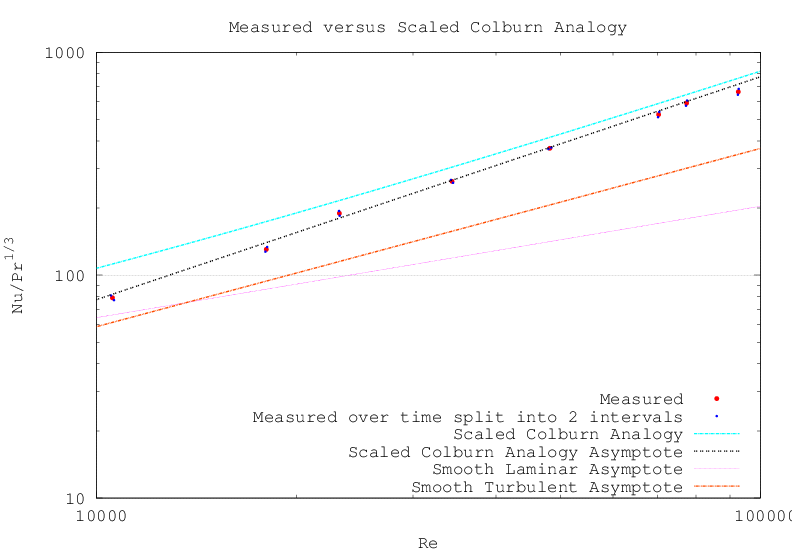The largest uncertainty in these measurements is wind-speed. Above 4 m/s (Re=80000) the anemometer is rated ± 3% accuracy, but the readings bounce around ± 10%. At lower speeds the readings are more stable, but accuracy drops quickly. The viscosity, pressure, and density of air may affect the relation between fan rotation rate and wind-speed in the tunnel, but their effects are not currently modeled.

It is clear from my fan calibration curves that wind-speed is not linear with fan rotation rate. I fit an exponential decay curve of the form: C⋅(1−σr).

Below Re=10000 the data is more complicated:Looking at the asymptotes, it is clear that the rough surface is not seeing laminar flow, which would produce more convection than the other flow types below Re=7000. Eliminating laminar flow from the smooth back surface model brought its simulation much closer to measured temperatures as well.

These points are interesting because they show less convection than expected. If they had shown more convection than expected, then it could have been due to mixing of natural and forced convection. But their showing less would appear to rule out the mixing of forced and downward natural convection.The rewritten section Scaled Colburn Analogy Asymptote describes the new correlation RST which brings most of the points into agreement as shown in the plot above.

There was a mistake in my simulation of the (insulated) back surface. It doesn't affect the measurement or simulation of the test surface. Here is a proper simulation of the plate with the rough side facing down: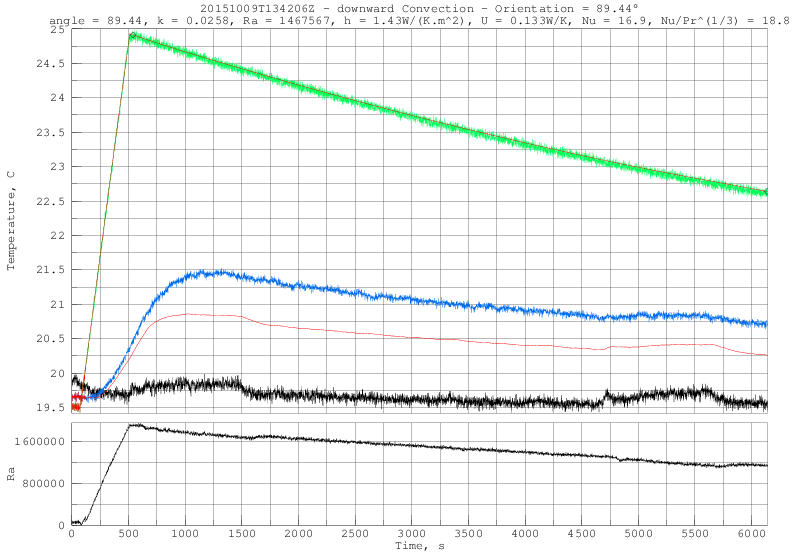The red lines are the simulations; the others are measured. The backside simulation expects more convection than was measured, resulting in the measured back face temperature being higher than its simulated temperature.

Because they are not thermally conductive over their whole surfaces, natural convection from the four vertical sides can't be modeled with established theory. My simulation models these sides as having 3.15 times the natural convection from the 52 mm × 305 mm metal surfaces not covered by insulation. The 3.15 factor was arrived at from natural convective runs on the Convection Machine.

The streamlines in Fujii and Imura's figure 14(f) show air from the edges of the plate moving toward the rising column at the plate's center. But in the Convection Machine this air has already been heated by the rough (downward) face and four sides. Thus the convection from the (upward) back side would be reduced and its temperature higher than if the other faces were not convecting.

With the rough test surface facing downward its convection is not affected by the other faces. With the plate 5 K hotter than ambient, convection from the bottom face is about 0.7 W and about 0.22 W for each vertical face. Their combined 0.93 W dwarfs the 0.32 W expected through the insulation for the back face (upward). So it is not surprising that the back face convection is reduced.

When the rough test surface faces up, its expected 2.3.0 W convection will experience reduction from the 1.2 W of convection from the other faces, complicating its analysis. More about this below.

With the current configuration, I am measuring the natural convection from a plate inclined a few degrees from facing downward. Turning the front tuning pegs shortens the support wires and tilts the plate.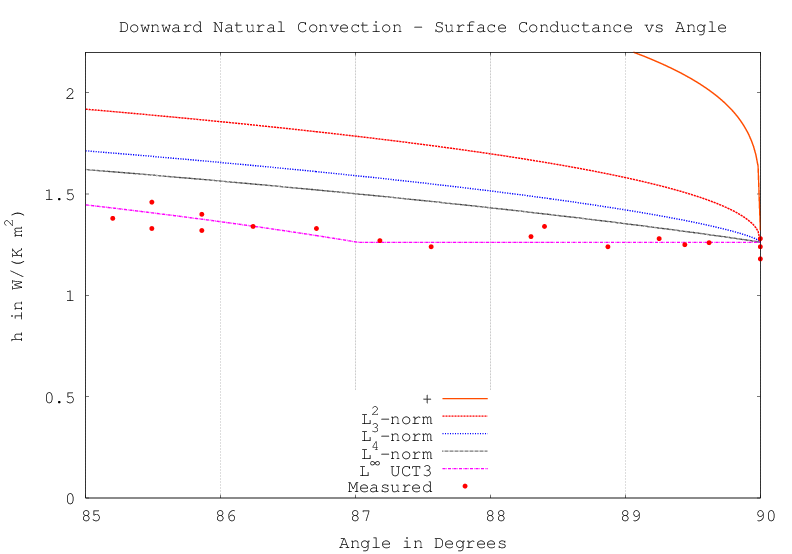This graph shows that downward convection does not blend with other convective modes. With θ between 87° and 90° downward convection is in control; between 0° and 87° the vertical mode is dominant. I have modified the UCT3 formula in the Downward Natural Convection section with this new knowledge.

 R = min(LH, LW)/2 h = k⋅max(Nu9.3(Ra(LH) cos θ)/LH , Nu45(Ra(R) sin θ)/R) 0°≤θ≤+90° UCT3

The random variation in the plot above makes it less convincing than it might be. I modified the firmware to increase the heating threshold from 5 K to 15 K, which increases the average ΔT from 3.7 K to 11 K and reduces the relative variation by about a factor of 3.Running the tilt tests at the higher temperature difference results in markedly reduced variation as can be seen by the proximity of the half-interval measurements (black dots) to the full-interval measurements (red dots). But the slope below 87.5° clearly does not match that derived from Nu9.3, the natrual convection from a smooth vertical plate! More about this later.

I also ran a series of forced convection trials with the 15 K peak temperature difference: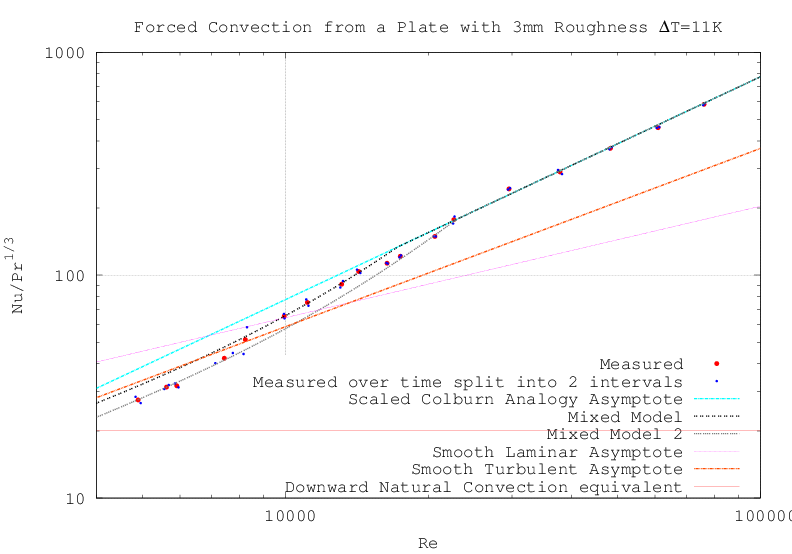There are several things to notice about this graph. The tests where only part of the plate had roughness penetrating the boundary layer seemed to have two distinct breakpoints: Re=16666 and Re=22222. For nearly all the measurements, the half-interval measurements (black dots) are very close to the full-interval measurements (red dots). There are two which are not so close. The black dots around Re=7440 lie close to the line, indicating the wind speed change which occured partway through the run. This can be seen in the bottom panel on page 8 of the PDF. The dots at Re=8237 had the same windspeed, perhaps indicating that the system spent time in both breakpoint modes.

Below Re=7500 the measurements track a slope of turbulent convection (Re4/5). Unfortunately the wind-tunnel can't produce wind speeds below 0.25 m/s (Re=4900), so we can't see the transition to natural downward convection.

Returning to natural convection, either the natural convection from a rough vertical surface differs from that of a smooth vertical surface or the formula combining the downward and vertical convection is wrong. The former can be tested by suspending the plate vertically. I plan to do this by wrapping the wire suspending the plate around its (insulated) back. In order to protect the insulation from being cut by the wire, a sheet of aluminum should be glued to the back. I was planning to do this already in order to test upward natural convection.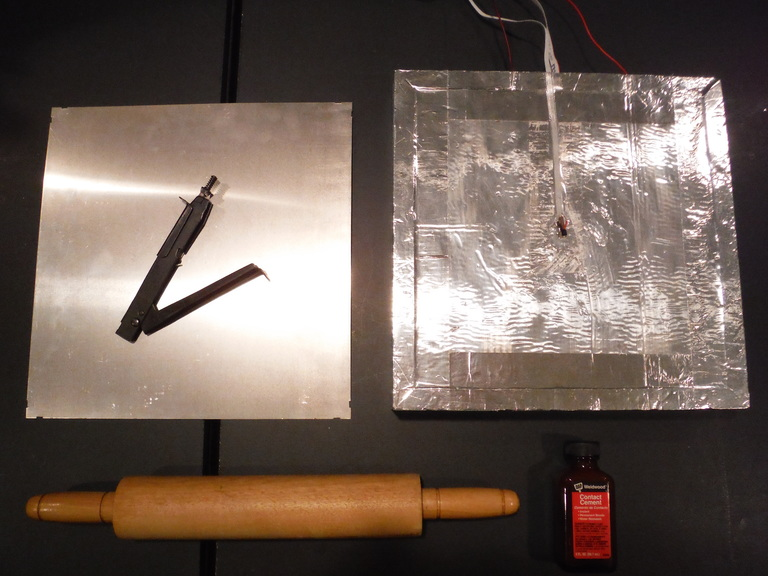Here is the back side of the plate ready to have the 0.65 mm sheet of aluminum glued to it. Notches have been cut (using the "nibbler" on the sheet) near the sheet corners to catch the wires which will suspend it.Gluing completed.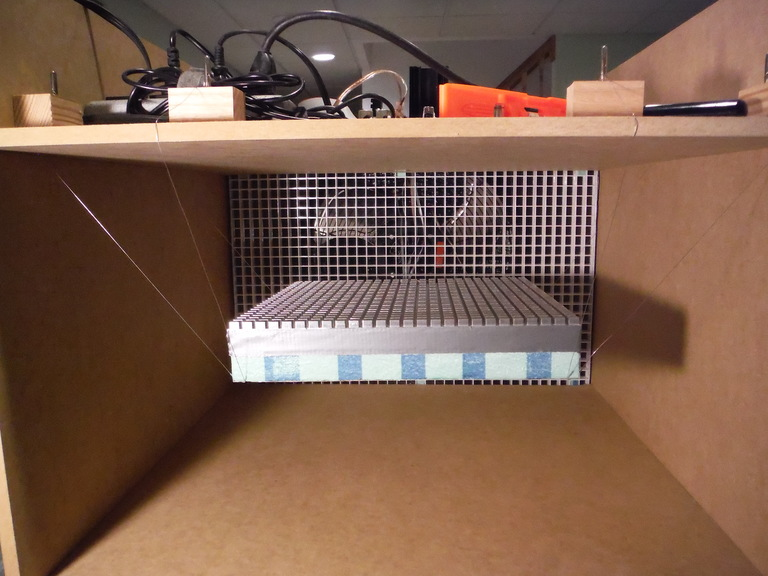Here the rough surface of the plate is facing up in the wind tunnel. But first I must rerun all the downward facing measurements.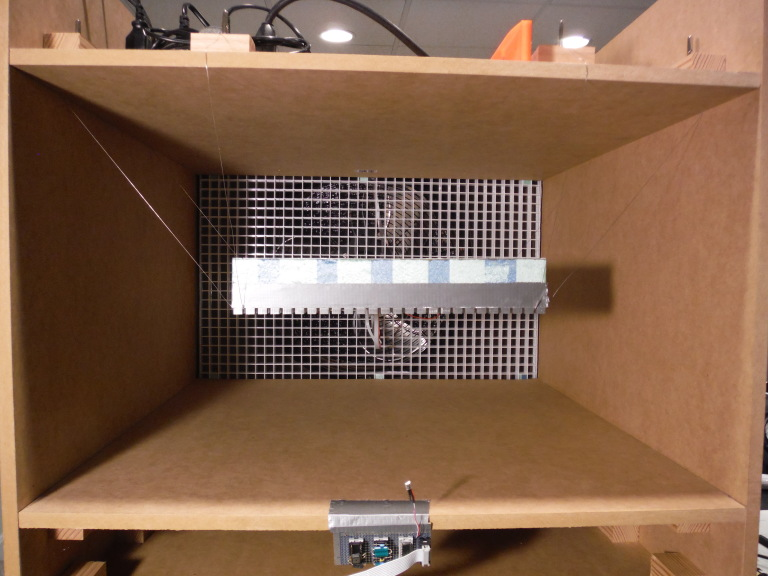The new back eliminated the dual modes seen earlier with peak ΔT=15K. Reducing the r/min to m/s conversion by 2% (which is within the ± 3% error band of the anemometer) and adjusting the breakpoint results in a beautiful fit!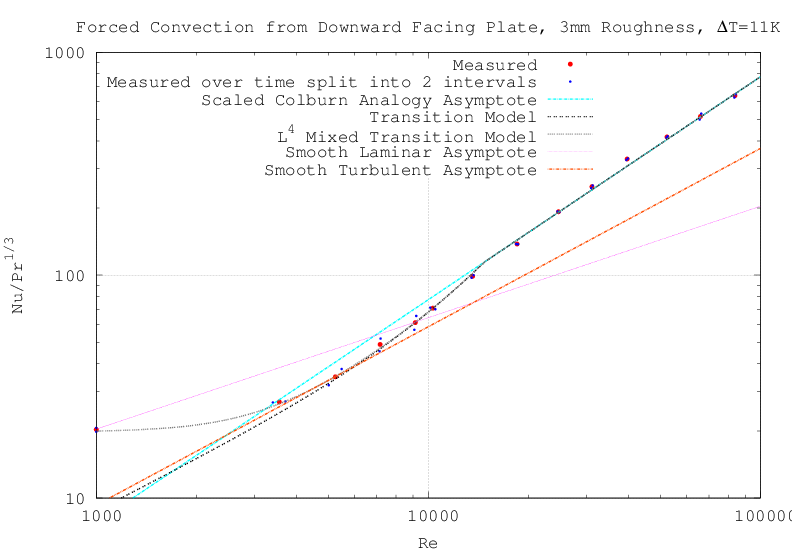The points at Re=3545 and Re=5231 could be following either the Smooth Turbulent Asymptote or the Transition Model; it's too close to tell. The reason that the Transition Model has less convection than the Smooth Turbulent Asymptote below Re=6000 is because the leading edge of the boundary layer is modeled as thinner than the roughness; but division by the local characteristic length, 0, is undefined at the leading edge.

As my blog post Upward Natural Convection details, there is no guarantee that natural convection of my plate with the rough surface facing up behaves in a manner which can be modeled. The air warmed by the insulated back and sides rises adjacent to the heated rough surface which draws air towards its center. So how strong is this effect? Measured with peak temperature differences of 15 K, 10 K, and 5 K, the measured upward natural convection is about half that predicted. But looking at the natural convection components, the deficit is nearly equal to sum of the side convective heat flows!

With the rough surface facing down, the mix of convective and radiative heat loss from the four sides matters little because both are subtracted from the overall heat loss. But when the rough surface faces upward it does matter; side convection reduces the rough surface convection while thermal radiation does not. Creating a plot of downward natural convection at a range of temperature differences allows evaluation of simulated mixtures. As the fraction of simulated radiative heat loss increases, the slope connecting the measured points increases. The graph below shows the fit when the effective radiative height of the side is 41% of its actual height, and the effective convective surface area is adjusted to fit:With this rough estimate of the relative strengths of convective and radiative heat loss, we are now ready to see whether the effect of the sides on the top surface can be reasonably modeled.

The plot below compares upward convection correlations with total non-radiative heat flow minus 93% of the (modeled) sides natural convection. There is less variation from point to point because upward natural convection is three times stronger than downward natural convection. "Horizontal Hot Top" is my generalization of the four conventional upward convection correlations.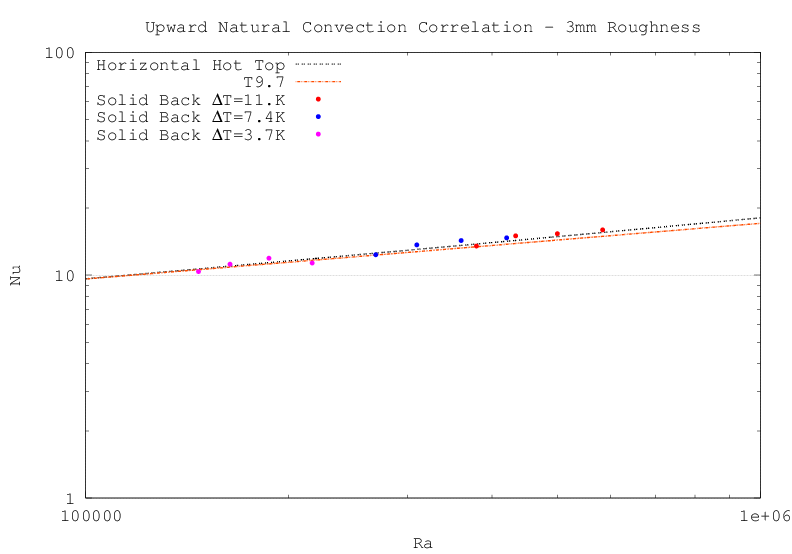The unexpectedly close match above lends support to the idea that air heated by the sides is drawn over the upper surface of the plate, reducing the effective temperature difference between the plate and air, and can be modeled as a reduction of upward convection by an amount proportional to the side convection.

Now that I have convection measurements at low fan speeds which are comparable in magnitude to natural convection, the next step is to evaluate mixed convection (with the rough surface facing upward).

Just as there was no guarantee of a workable model for the interaction of the sides with the top in still air, there is no guarantee of a model of that interaction in forced air. Needed is a generalization matches the model developed for V=0 and matches the forced correlation as V grows.

The graph below shows the correlation I have arrived at for upward convection (see simulations). It assumes that mixed convection for the four short sides is the L4-norm of the natural and forced convections and that 93% of only the natural component of the side convection is absorbed by the convection of the (upward-facing) rough surface.If the L4-norm mixing for the short sides is instead L2, the increase in natural convection from the sides reduces the top surface convection, spoiling the upward-convection match with L2-norm (gray dashed line) and other L-norm exponents (best is about L2.3).

Here are the calculated values of convection from all six sides of the plate at windspeeds from 0 to 4 m/s and ΔT=11K:

 insulatedback parallelside windwardleeward total rough windspeed + 2 ⋅ + 2 ⋅ = vs @ 53.1mW/K 55.3mW/K 55.3mW/K 0.272W/K 0.467W/K 0.0m/s 55.2mW/K 55.4mW/K 55.4mW/K 0.274W/K 0.484W/K 0.12m/s 60.4mW/K 55.5mW/K 55.4mW/K 0.280W/K 0.520W/K 0.25m/s 65.0mW/K 55.9mW/K 55.9mW/K 0.286W/K 0.666W/K 0.50m/s 68.5mW/K 57.3mW/K 57.6mW/K 0.296W/K 1.18W/K 1.0m/s 71.0mW/K 61.5mW/K 63.4mW/K 0.318W/K 2.22W/K 2.0m/s 72.6mW/K 72.4mW/K 78.4mW/K 0.371W/K 4.36W/K 4.0m/s

I had expected L4-norm mixing for upward convection. So these results will require modifications to my theory of mixed convection.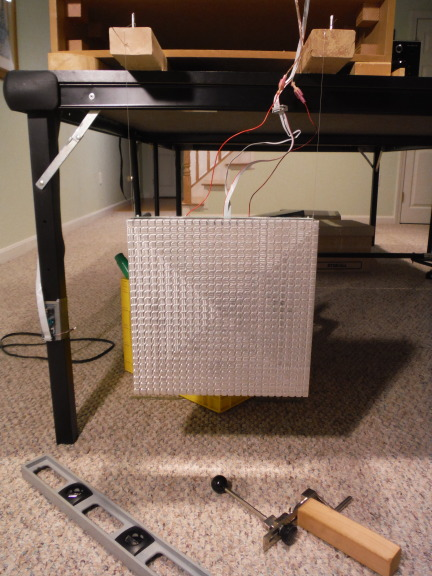Unexpected results for downward convection at small-angles raised the question of whether vertical natural convection is the same for rough and smooth plates. This photo shows the plate suspended vertically by steel wire from the two boards above. The ambient temperature sensor is taped to the table leg. I measured the natural convection over three temperature ranges as was done in the other natural convection runs.

If there is less convection than expected, then it could be due to heat from one side reducing the convection of a side above it, as happens in the upward facing case.

But slightly more convection than expected was measured. As the plate is no longer in the wind tunnel, modeling the emissivity of the room as 0.9 (versus 0.8 for the wind tunnel) brings the simulation into reasonable agreement with measurement. It thus appears that, at least for laminar flows from rectangular plates, natural convection from a rough surface has the same magnitude as convection from a smooth surface.

The graph below is linked to a pdf of the measurements and simulations of natural convection in level and vertical orientations.## Future Experiments• The results for downward convection do not discern whether mixing is L4-norm or higher (L-norm is the max function). I have bought a solid-state relay which the micro-controller will use to operate the fan at speeds low enough to answer this question.